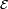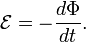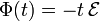# Maxwell (unit)

In physics, the maxwell (symbol Mx) is the unit of magnetic flux in the Gaussian and emu (electromagnetic) systems of units, which both are cgs (centimeter-gram-sec) systems of units. The relation to the SI unit weber (Wb) is,

1 Mx ≡ 1 abVs = 10−8 Wb.

The name of the unit honors James Clerk Maxwell, who formulated a unified theory of electromagnetism.

In the emu system the maxwell is a derived unit (abampere is an emu base unit), in the Gaussian system the maxwell is a base unit.

## Definition

The definition is based on Faraday's law for magnetic induction. This law connects electromotive force$\mathcal{E}$, (in abV = 10−8 V), to a rate of change of magnetic flux Φ (in Mx/s).$\mathcal{E} = -\frac{d\Phi}{dt}.$

If Φ is linear in time—i.e., Φ changes with a uniform rate—and if Φ = 0 at t = 0, then$\Phi(t) = - t\, \mathcal{E}$

This equation forms the basis of the definition, which reads: One maxwell is the magnetic flux which, linking a circuit of one turn (flux through one loop), would produce in it an electromotive force of one abvolt if it were reduced to zero at a uniform rate in one second.

Clearly, the dimension of Mx is abV⋅s = 10−8 V⋅s. The definition of the SI unit of flux, weber, is almost the same as that of maxwell, with volt replacing abvolt. Hence one maxwell corresponds to 10−8 weber.

The maxwell and its definition were adopted by the Fifth International Electrical Congress held in Paris in 1900. It was reconfirmed when the Advisory Committee on Nomenclature of the International Electrotechnical Commission adopted the name maxwell for the unit of magnetic flux at its meeting in Stockholm in 1930.